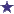# IV Compounding Math Question: How is a Tic Tac Toe Board Used In This Example?

by Sherri
(Raleigh NC USA)An order for 6 liters of 0.9% soium chloride solution is to be prepared from 23.4% concentrated NaCl solution and sterile water for injection.How many mL of each will be used?

The answer is supposed to be 231 mL of 23.4% and 5769 mL of water but I can't seem to figure out how they got their answer or what formula they used. What is the tic tac toe board supposed to look like? Do I multiply by 6, 6000, or 1000? or do i do the usual cross multiply and divide with the 1000?

### Comments for IV Compounding Math Question: How is a Tic Tac Toe Board Used In This Example?

Average RatingRatingTic Tac Toe Board and Answer... by: Robert This Pharmacy IV Math question can be solved by using the Tic Tac Toe Board. Using the amounts supplied, the board would be set up as follows: Middle square – 0.9Top left square – 23.4Bottom left square – 0 Following the instructions, subtract the amount in the middle square from the top left square – 23.4% - 0.9% = 22.5% - and this amount goes in the bottom right square. Do the same thing with the middle left and bottom left square – 0.9% - 0 = 0.9% and put this amount in the top right square. Next add the top right and bottom right numbers – 22.5 + 0.9 = 23.4. The answers are then multiplied by the volume needed, which is 6 liters or 6000 milliliters.. 6000 multiplied by 0.9 divided by 23.4 equals 231 ml of concentrated NACL. 6000 – 231 = 5769 ml of sterile water. Here is an example of how to set up a Tic Tac Toe Board to aid in finding the answer to solution preparation. Use a regular Tic Tac Toe Board that you can simply draw on a piece of paper. In the middle square of the board write the desired strength. In the top left square, place the higher strength solution. In the bottom left square, place the lower strength unless you are using water in which case this number would be a zero. Subtract the amount in the middle square from the amount in the top left square. Put this number in the bottom right square, then subtract the amount in the middle square from the bottom left square and put this amount in the top right square. Add the numbers in the top and bottom right squares and divide the top right number and the bottom right number by this amount. Multiply each answer by the volume needed and the result will be how much is needed of each solution. To make certain it is the right answer, add the two and it should be equal to the total volume. You will find the majority of the time the Tic Tac Toe Board will help you to find the right solution. Because practicality dictates that it is easier to dilute than to compound preparations, alligation is the method used for solving these problems when mixing different strength products. Using a Tic Tac Toe Board for the process is one of the simplest ways of doing so.

 RatingI hope this solution will help you. by: Anonymous 1. 23.4%-0.9% = 22.5%2. 0.9%-0=. 0.9%Total quantity you get is 0.9+22.5= 23.4So 6000ml of 0.9 is 6000*0.9\23.4= 231 ml231ml is concentrated NACL solution. To find out sterile water we subtract 6000ml - 231 ml= 5769 ml sterile water.